# Think Like a Database Designer Before Creating an Excel Dashboard Chart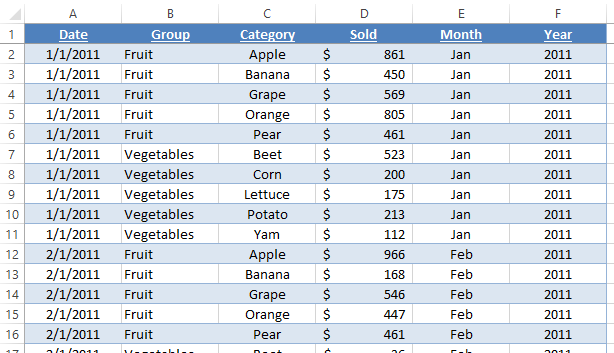Recently, a fan asked for some help.  However, when I got their file, I saw that they needed some help with basic concepts, so I thought I would share those concepts with you.

Now, let me preface that I am not a master database or Excel designer, so I am sure that I also have a lot to learn, but perhaps I can save you some time with this technique.

If you want to create dynamic dashboard charts in Excel like this: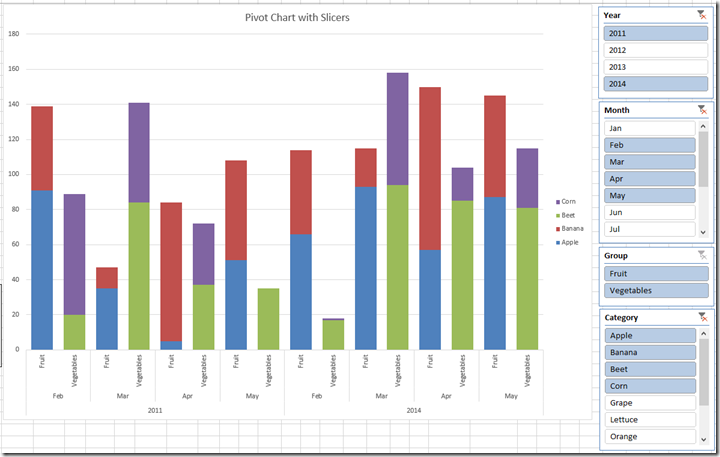you have to make sure you spend some time setting up your data structure.

Here is the issue with the data file that I received, they had placed their data for each year and month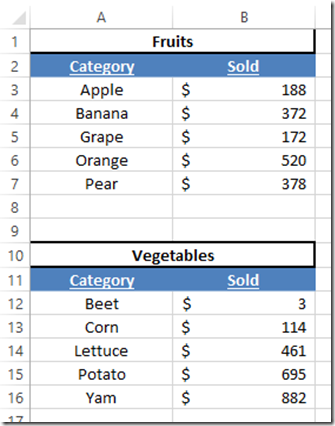on separate Excel worksheet tabs.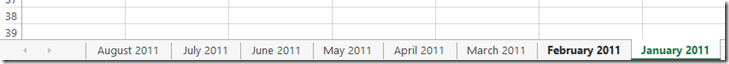This makes your job as an Excel Dashboard Designer very very difficult.  Why you may ask?  Because it is very difficult to gather this data without very complex formulas.  Way too much work if you ask me.  Also, it will eventually cause you problems as time goes on.

So what should I do instead?

Lets think like a database designer.

They don’t create a table for every month of data.  They simply put the data into one large table if feasible instead of breaking up the data into different worksheets.

Keep combining your data as much as you can.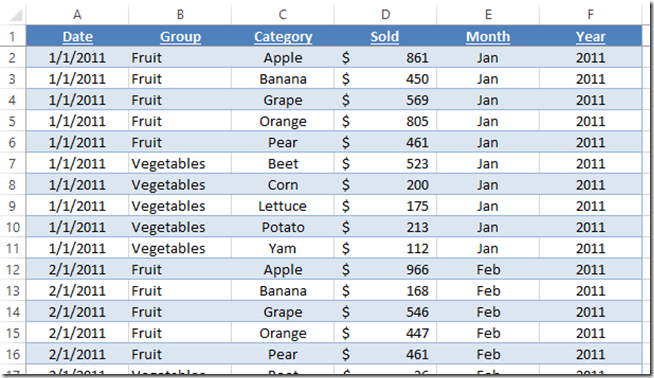There is no reason to break up this data into multiple worksheet tabs.  I created calculated columns of data on Month and Year to create extra filters and sorts instead of creating new tabs.  You should consider simplifying your data in such a way.

Once again, I didn’t say I was a master, so make sure and leave your comments below on how we could make this even better or other issues that new Excel dashboard designers face in the comments below.

In the next few posts, we will expand on this concept to make an Excel Dynamic Dashboard Pivot Chart with Slicers.

# If you found the website and tutorials helpful, please consider donating to keep the lights on.

## Donate with PayPal here:Steve=True

1.Andrew

Hey – this is great advice, but my question is – is there simple way of combining the data into a single table, or is it unfortunately a laborious copy and paste job?
Andrew

•SteveEqualsTrue

Thanks Andrew. For your first time, I would imagine that it would be best to do it manually. If you have multiple, you can create a macro to do the heavy lifting for you. Steve=True

•Jose R.

Hi Andrew

If you have to do this task multiple times (sometimes the spreadsheets “need” to have multiple tabs due to different reasons/functionality) the best way is to write a VBA code and run it when needed.

Here is a small example of a code to go through multiple spreadsheets and copy data to a single spreadsheet (order spreadsheets tabs so the summary and manipulation spreadsheets show up first in the workbook):

Sub SummaryTable()

‘Creates one table with data from multiple spreadsheets
‘Jose Relvas (06.01.2016)

Dim i As Integer
Dim j As Integer
Dim k as Integer
Dim Wrbsheets as integer
Dim Summary As Worksheet

Set Summary = Worksheets(“Summary”) ‘writes data in a spreadsheet named Summary. Change name if your spreadsheet has a different name

Wrbsheets = Thisworkbook.Worksheets.Count

Summary.Range(“A2:Z10000”).ClearContents

j = 2 ‘1st row in Summary where we want to write data to

For k = 3 to Wrbsheets ‘change initial k to the 1st spreadsheet number where data is to be copied. in this case I assume spreadsheets 1 and 2 have no data I need to copy

For i = 14 To 500 ‘change initial i to the 1st row where there is data to be copied and final i to last row where there is data. the bigger the value more time is needed to calculate

If IsEmpty(worksheets(k).Cells(i, 2).Value) = True Or worksheets(k).Cells(i, 2).Value = “Budget” Then ‘change conditions to look for empty cells. If none remove the If from the code

Else ‘Cells(j,1) means row j, column 1 (same as column A) where j is the number of each iteration. starts at 2 in this case and is incremented by 1 everytime a row of data to be copied is found

Summary.Cells(j, 1).Value = worksheets(k).Cells(i, 1).Value
Summary.Cells(j, 2).Value = worksheets(k).Cells(i, 2).Value
Summary.Cells(j, 3).Value = worksheets(k).Cells(i, 3).Value
Summary.Cells(j, 4).Value = worksheets(k).Cells(i, 4).Value
Summary.Cells(j, 5).Value = worksheets(k).Cells(i, 6).Value
Summary.Cells(j, 6).Value = worksheets(k).Cells(i, 7).Value
Summary.Cells(j, 7).Value = worksheets(k).Cells(i, 8).Value
Summary.Cells(j, 8).Value = worksheets(k).Cells(i, 9).Value
Summary.Cells(j, 9).Value = worksheets(k).Cells(i, 10).Value
Summary.Cells(j, 10).Value = worksheets(k).Cells(i, 11).Value
j = j + 1
End If
Next i
Next k

MsgBox “Database created”, vbInformation, “Dashboard” ‘message box informing when operation is finished

End Sub

•SteveEqualsTrue

Thanks Jose!